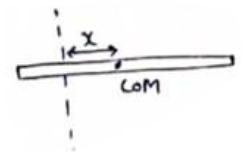Deepak Scored 45->99%ile with Bounce Back Crack Course. You can do it too!

# Solve the following :

Question:

The moment of inertia of a uniform rod of mass $0.50 \mathrm{~kg}$ and length $1 \mathrm{~m}$ is $0.10 \mathrm{~kg}-\mathrm{m}^{2}$ about a line perpendicular to the rod. Find the distance of this line from the middle point of the rod.

Solution:By parallel axis theorem,

$I_{a x i s}=I_{C O M}+m x^{2}$

$I_{a x i s}=\frac{m L^{2}}{12}+m x^{2}$

$0.1=\frac{(0.5)(1)^{2}}{12}+(0.5) x^{2}$

$X=0.34 m$